Next: About this document ... Up: lab_template Previous: lab_template

Subsections

# Cylindrical and Spherical Coordinates

## Getting Started

To assist you, there is a worksheet associated with this lab that contains examples and even solutions to some of the exercises. You can copy that worksheet to your home directory with the following command, which must be run in a terminal window for example, not in Maple.

cp /math/calclab/MA1024/Coords_start.mws ~/My_Documents


You can copy the worksheet now, but you should read through the lab before you load it into Maple. Once you have read to the exercises, start up Maple, load the worksheet Coords_start.mws, and go through it carefully. Then you can start working on the exercises.

## Background

Defining surfaces with rectangular coordinates often times becomes more complicated than necessary. A change in coordinates can simplify things. Cylindrical coordinates can simplify plotting a region in space that is symmetric with respect to the-axis such as paraboloids and cylinders. The paraboloid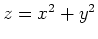would become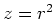and the cylinder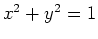would become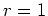. Spherical coordinates would simplify the equation of a sphere, such as, to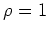. The conversion tables below show how to make the change of coordinates.

To change to cylindrical coordinates from rectangular coordinates use the conversion: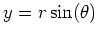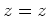Where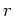is the radius in the x-y plane and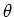is the angle in the x-y plane.

To change to spherical coordinates from rectangular coordinates use the conversion: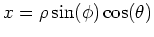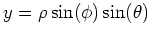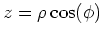Whereis the angle in the x-y plane;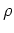is the radius from the origin in any direction; and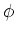is the angle in the x-z plane.

Using a triple integral to find the volume of a solid translates in the following manner:## Exercises

1. Given the rectangular equation for a circular cone:A)
Graph the equation using the domain values of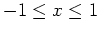,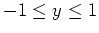and the range values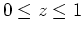.
B)
Write the equation in cylindrical coordinates and then graph the equation.
C)
Write the equation in spherical coordinates and graph it.
D)
Use rectangular coordinates and a triple integral to find the volume of a right circular cone of height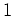. Now repeat this using cylindrical coordinates. Which method is easier?

2. Now suppose an ice cream cone is bounded below by the same equation of the cone given in exercise 1 and bounded above by the sphere. Plot the ice cream cone using rectangular coordinates over the same intervals as exercise 1a. Find the volume of the ice cream cone using spherical coordinates.Next: About this document ... Up: lab_template Previous: lab_template
Dina Solitro
2008-04-23